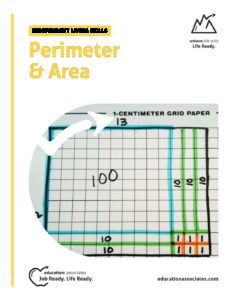# Perimeter & Area

Perimeter & AreaHow much fence do you need for the dog run? How much grass seed do you need for a yard? How much carpet to buy for a room? With this program, students will learn how to easily calculate perimeter and area and how to use these calculations to solve different real-life problems.

Competencies include:

• Define Key Vocabulary Words
• Explain How to Determine the Perimeter of a Square, Triangle and Rectangle
• Explain How to Determine the Area of a Square, Triangle and Rectangle
• Use Correct Formulas to Solve Real-World Problems

MM1002SL